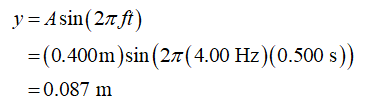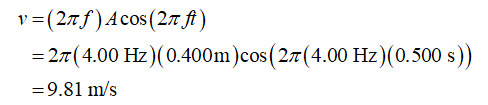# In a spring block system the oscillation frequency is 4.00 Hz. If the amplitude of oscillation is 0.400 m, determine the position, speed and acceleration at t = 0.500 s.

Question
11 views

In a spring block system the oscillation frequency is 4.00 Hz. If the amplitude of oscillation is 0.400 m, determine the position, speed and acceleration at t = 0.500 s.

check_circle

The position,The equation for speed...

### Want to see the full answer?

See Solution

#### Want to see this answer and more?

Solutions are written by subject experts who are available 24/7. Questions are typically answered within 1 hour.*

See Solution
*Response times may vary by subject and question.
Tagged in